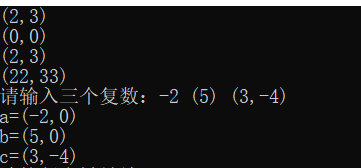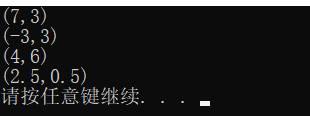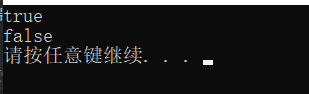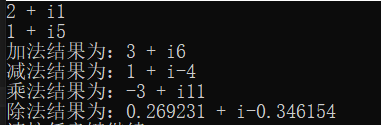• 简单的Complex复数运算，初学者可以参考下！！！
• ## Complex复数类

千次阅读 2017-09-12 20:46:02
complex复数类，进行加减乘除操作。
public class Complex {
private double RealPart;//实部
private double ImagePart;//虚部

/* 定义构造函数 */
public Complex(){
}

/* 含参构造方法*/
public Complex(double realPart, double imagePart){
this.RealPart = realPart;
this.ImagePart = imagePart;
}

public void setRealPart(double realPart){
this.RealPart = realPart;
}

public void setImagePart(double imagePart){
this.ImagePart = imagePart;
}

public double getRealPart(){
return RealPart;
}

public double getImagePart(){
return ImagePart;
}

/* 复数的加法 */
double realPart1 = this.getRealPart() + o1.getRealPart();
double imagePart1 = this.getImagePart() + o1.getImagePart();
return new Complex(realPart1,imagePart1);
}

/* 复数的减法 */
public  Complex subtract(Complex o1){
double realPart1 = this.getRealPart() - o1.getRealPart();
double imagePart1 = this.getImagePart() - o1.getImagePart();
return new Complex(realPart1,imagePart1);
}

/* 复数的乘法 */
public Complex multiply(Complex o1){
double realPart1;
double imagePart1;
if(this.getImagePart() != 0 && o1.getImagePart() != 0){
realPart1 = (this.getRealPart() * o1.getRealPart()) - (this.getImagePart() * o1.getImagePart());
imagePart1 = (this.getRealPart() * o1.getImagePart()) + (this.getImagePart() * o1.getRealPart());
}
else{
realPart1 = (this.getRealPart() * o1.getRealPart());
imagePart1 = (this.getRealPart() * o1.getImagePart()) + (this.getImagePart() * o1.getRealPart());
}
return new Complex(realPart1,imagePart1);
}

/* 复数的除法 */
public Complex divide(Complex o1){
double realPart1;
double imagePart1;
realPart1 = (this.getRealPart() * o1.getRealPart()) + (this.getImagePart() * o1.getImagePart()) / (o1.getImagePart() * o1.getImagePart() + o1.getRealPart() * o1.getRealPart());
imagePart1 = (this.getImagePart() * o1.getRealPart()) - (this.getRealPart() * o1.getImagePart()) / (o1.getImagePart() * o1.getImagePart() + o1.getRealPart() * o1.getRealPart());
return new Complex(realPart1,imagePart1);
}

public String toString(){
return this.RealPart+" + "+this.ImagePart+"i";
}
}
展开全文java
• C++ complex复数类用法详解 <C++ durationC++ complex> 转自 http://c.biancheng.net/view/703.html 复数是 a+bi 形式的数，其中 a 和 b 是真数，在C++代码中是浮点值，i 是根号 -1。a 被称作复数的实数...
C++ complex复数类用法详解
< C++ durationC++ complex >
转自   http://c.biancheng.net/view/703.html
复数是 a+bi 形式的数，其中 a 和 b 是真数，在 C++ 代码中是浮点值，i 是根号 -1。a 被称作复数的实数部分，b 乘以 i 被称作虚数部分。 使用复数的程序一般都很专业，例如，复数可以用于电气和电磁理论、数字信号处理，当然也可以用于数学。复数可以用来生成非常复杂的 Mandelbrot 集合和 Julia 集合的分形图。 complex 头文件定义了用于处理复数的功能。complex<T> 模板类型的实例表示的是复数，这里定义了 3 个特化类型：complex<float>、complex<double>、complex<long double>。在这一节中，全部使用 complex<double>，但其他特化类型的操作是基本相同的。
生成表示复数的对象
complex<double> 类型的构造函数接受两个参数，第一个参数是实部的值，第二个部分是虚部的值。例如：

std::complex<double> z1 {2, 5}; // 2 + 5istd::complex<double> z; // Default parameter values, are 0 so 0 + 0i
它也有拷贝构造函数，因此可以按如下方式复制 z1:

std::complex<double> z2 {z1}; // 2 + 5i
显然，我们需要复数常量以及复数对象，命名空间 std::literals::complex_literals 中定义了 3 个运算符函数，在这个命名空间中，命名空间 literals 和 complex_literals 都是内联定义的。在对  std::literals::complex_literals、std::literals 或 std::complex_literals 使用 using 指令之后，就可以访问用于复数常量的运算符函数。假设使用了一个或多个这种指令，并且 using std::complex 对这一节余下的代码都有效。 运算符 ""i() 函数定义了实部为 0 的 complex<double> 类型的常量。因此，3i 是一个等同于 complex<double>{0，3} 的常量。当然，可以用实部和虚部表示复数。例如：

z = 5.0 + 3i; // z is now complex<double>{5, 3}
这展示了如何定义两部分都是非零值的复数，并顺便说明已经为复数对象实现了赋值运算符。可以对 complex<float> 常量使用后缀if，对 complex<long double> 常量使用后缀il，例如 22if 或 3.5il。这些后缀是由函数 operator""if() 和 operator""il() 定义的。注意，不能写成 1.0+i 或 2.0+il，因为这里的 i 和 il 会被解释为变量名，必须写成 1.0 +li 和 2.0+1.0il。 所有的复数类型都定义了成员函数real()和imag()，它们可以用来访问对象的实部或虚部，或者用提供的参数设置这些部分。例如：

complex<double> z{1.5, -2.5}; // z: 1.5 - 2.5iz.imag(99); // z: 1.5 + 99.0iz.real(-4.5); // z: -4.5 + 99.0istd::cout << "Real part: " << z.real()<< " Imaginary part: " << z.imag()<< std::endl;// Real part: -4.5 Imaginary part: 99
real() 和 imag() 接受参数的版本什么都不会返回。 有为复数对象实现流的插入和提取的非成员函数模板。当从流中读取一个复数时，它可能只有实部，例如 55，或者括号中只有实部，例如(2.6)，或者实部和虚部在由一个逗号隔开的括号中，例如(3,-2)。如果只提供了实部，虚部会为 0。下面是一个示例：

complex<double> z1, z2, z3; // 3 default objects 0+0istd:: cout << "Enter 3 complex numbers: ";std::cin >> z1 >> z2 >> z3; // Read 3 complex numbersstd::cout << " z1 = " << z1 <<" z2 = " << z2 << " z3 = "<< z3 << std::endl;
下面是示例的输入和输出结果：
Enter 3 complex numbers: -4 (6) (-3, 7) z1 = (-4,0) z2 = (6,0) z3 = (-3,7)
如果输入的一个复数没有括号，就不会有虚部。但是，在括号中可以省略虚部。复数的输出周围总是有括号，虚部即使为 0 也会被输出。
复数的运算
complex 类模板为有复数操作数的二元运算符 +、-、*、/ 及一元 + 和 - 运算符定义了非成员函数。成员函数定义了 +=、-=、*= 和 /=。下面是使用它们的一些示例：

complex<double> z {1,2}; // 1+2iauto z1 = z + 3.0; // 4+2iauto z2 = z*z + (2.0 + 4i); // -1+8iauto z3 = z1 - z2; // 5-6iz3 /= z2; // 815385-0.523077i
注意，复数对象和数值常量之间的运算需要数值常量是正确的类型。不能将整数常量加到 complex<double> 对象上；为了能够进行这个运算，必须写成 2.0。
复数上的比较和其他运算
一些非成员函数模板可以用来比较两个复数对象相等或不相等。也有 == 和 !=运算可以用来比较复数对象和数值，这里数值会被看作虚部为 0 的复数。为了相等，所有的部分都必须相等，如果操作数的实部或虚部不同，它们就不相等。例如：

complex<double> z1 {3,4}; // 3+4icomplex<double> z2 {4,-3}; // 4-3istd::cout << std::boolalpha<<(z1 == z2) << " " // false<< (z1 != (3.0 + 4i)) << " " // false<< (z2 == 4.0 - 3i) << '\n'; // true
注释中的结果很清楚。注意在最后一个比较中，编译器会将 4.0-3i 看作复数。 另一种比较复数的方法是比较它们的量。各部分值和复数的实部及虚部都相同的向量的量和复数相同，是两部分平分和的平方根。非成员函数模板 abs() 接受 complex<T> 类型的参数，并返回一个T类型的量。下面是一个将 abs() 函数应用到前面的代码段中定义的 z1 和 z2 上的示例：

std::cout << std::boolalpha<< (std::abs(z1) == std::abs(z2)) // true<< " " <<std::abs(z2 + 4.0 + 9i); // 10
最后的输出值是 10，因为作为 abs() 的参数的表达式的计算结果是 (8.0+6i)；82 和 62 是 100，平方根是 10。
norm() 函数模板会返回复数的量的平方。arg() 模板会返回以弧度为单位的相角，是复数 z 对应的 std::atan(z.imag()/z.real())。conj() 函数模板会返回共轭复数，是 a+bi 和 a-bi。polar() 函数模板接受量和相角作为参数，并返回和它们对应的复数对象。prqj() 函数模板返回的复数是复数参数在黎曼球上的投影。
一些非成员函数模板提供了一整套的三角函数，并为复数参数提供了双曲函数。也有用于复数参数的 cmath 版本的函数 exp()、pow()、log()、log10() 和 sqrt()。下面是一个有趣的示例：
纯文本复制

complex<double> zc {0.0, std::acos(-1)};std::cout << (std::exp (zc) +1.0) << '\n'; // (0, 1.22465e-16) or zero near enough
acos(-1) 是 π，所以这揭示了欧拉方程令人震惊的真相，π 和欧拉数 e 是有关联的：eiπ+1=0。
展开全文• C++写的complex复数的代码 里面包含头文件、test文件、执行文件 便于初学者了解声明与定义
• C++程序设计 Complex复数类 直角坐标系 包括复数间的加、减、乘、除，还有两个复数间的夹角
• C++封装的Complex复数类（源代码），基本完整封装了所有的操作，包括标准的输入和输出。主要作为参考代码。
• Java实现Complex复数类的加减乘除 实现Complex类的成员以及构造方法还有get( )和set（）方法 进行加法减法的编写，确定加法和减法的参数 以及方法的public protected 和 private的属性 对乘法除法进行相同的思路...
Java实现Complex复数类的加减乘除
实现Complex类的成员以及构造方法还有get( )和set（）方法进行加法减法的编写，确定加法和减法的参数 以及方法的public protected 和 private的属性对乘法除法进行相同的思路分析还有对to String ()方法进行重写对equals（）方法进行重写，使之成为判断Complex类的相等判断方法接下来看一看我们的构造方法以及我们的重写的方法
public class Complex {
private  double real;
private  double vir;

public Complex(double real,double vir) {
this.real=real;
this.vir=vir;
}
public Complex() {
this(0.0,0.0);

}
public Complex(Complex c) {
this(c.real,c.vir);
}
public Complex(double vir) {
this.real=0.0;
this.vir=vir;
}
public double getReal() {
return real;
}
public void setReal(double real) {
this.real = real;
}
public double getVir() {
return vir;
}
public void setVir(double vir) {
this.vir = vir;
}
}


看看我们写的重写方法：
public boolean equals(Object obj) {
if(null==obj) {						//传入的对象为空
return false;
}
if(!(obj instanceof Complex)) {		//不是同一种类
return false;
}
if((this == obj)) {					//两个首地址直接是相等的 ，两个类相等
return true;

}
Complex c=(Complex) obj;
return Math.abs(this.real-c.real)<1e-6 && Math.abs(this.vir-c.vir)<1e-6;
}

这里穷尽了实部的虚部的各种情况，进行合理的输出：
public String toString() {
if(this.vir ==0&&Math.abs(real)>1e-6) {
return real+"";
}
if(this.vir==1&&Math.abs(real)>1e-6) {
return real+"+"+"i";
}
if(vir<0&&Math.abs(real)>1e-6) {
if(vir==-1) {
return real+"-"+"i";
}
return real+"-"+(-vir)+"i";
}
if(Math.abs(real)<10e-6&&Math.abs(vir)<1e-6) {
return "0.0";
}
if(Math.abs(real)<10e-6&&vir==-1 ) {
return "-i";
}
if(Math.abs(real)<10e-6&&vir==1 ) {
return "i";
}
if(Math.abs(real)<10e-6) {
return vir+"i";
}
return real+"+"+vir+"i";
}


	public void  add(Complex one) {
this.vir+=one.vir;
this.real+=one.real;
}
public static Complex add(Complex one,Complex two) {
one.vir+=two.vir;
one.real+=two.real;
return one;
}

接下来进行减法的设计，和加法同理，不过我们想调用工具，于是做一个求相反数的类。


public static Complex sub(Complex one,Complex two) {
}

public static Complex opposite(Complex one) {
return new Complex(-one.real,-one.vir);
}

乘除与加减类似，我们打算用一个求倒数的方法来让我们调用：
public  static Complex multiply(Complex one,Complex two) {
double c=one.real;
one.real=one.real*two.real-two.vir*two.vir;
one.vir=c*two.vir+one.vir*two.real;
return one;
}

public static Complex reciprocal(Complex a) {
int model =(int) (a.real*a.real+a.vir*a.vir);
if(Math.abs(model)<10e-6)
return null;
else {
a.real=a.real/model;
a.vir=a.vir/model;
return a;
}
}
public static Complex divide(Complex one,Complex two) {
Complex c=reciprocal(two);
return  c==null ?null: multiply(one,c);
}


这样我们就设计好了一个类，虽然不算完备，但已经实现了该有的加减乘除方法。 也具备一定的工具思想！
展开全文Java
• ## C++complex复数类

千次阅读 2020-03-31 20:21:40
复数是形如a+bi的数，复数由实部和虚部构成，在C++的模板库中由complex类，可以直接调用，包含在complex头文件中。
  复数是形如a+bi的数，复数由实部和虚部构成，在C++的模板库中由complex类，可以直接调用，包含在complex头文件中，再使用时应该添加#include<complex>。下面介绍一些基本操作： 生成复数对象   complex类型的构造函数接受两个参数，第一个参数是复数实部的值，第二个参数是虚部的值。要想生成一个复数对象，并且对其值进行修改，参考以下代码：
#include<iostream>
#include<complex>

using namespace std;

int main()
{
complex<double>z(2, 3);
complex<double>z1;
complex<double>z2(z);

cout << z << endl;
cout << z1 << endl;
cout << z2 << endl;
z.real(22);
z.imag(33);//修改z的实部和虚部的值
cout << z << endl;

complex<double>a, b, c;
cout << "请输入三个复数：";
cin >> a >> b >> c;
cout << "a=" << a << endl;
cout << "b=" << b << endl;
cout << "c=" << c << endl;
system("pause");
return 0;
}

结果如下，程序实现了复数的生成，实部和虚部的修改，或者是按照用户需要生成复数复数的运算   复数和实数一样都有加减乘除四则运算，这些运算符号在complex模板中已经被重载过，能够直接使用，下面代码示例展示了其功能：
#include<iostream>
#include<complex>

using namespace std;

int main()
{
complex<double> z(2 , 3);
complex<double> z1 = z + 5.0;
cout << z1 << endl;
complex<double> z2 = z - 5.0;
cout << z2 << endl;
complex<double> z3 = z*2.0;
cout << z3 << endl;
complex<double>z4 = z / complex<double>(1,1);
cout << z4 << endl;
system("pause");
return 0;
}复数的比较   复数的比较需要同时比较实部和虚部是否都相等，有其一不等两数就 不等，下面是示例代码：
#include<iostream>
#include<complex>

using namespace std;

int main()
{
complex<double> z1(2, 3);
complex<double> z2(3, 4);
complex<double> z3(2, 3);
cout << boolalpha << (z1 == z3) << endl;
cout << boolalpha << (z1 == z2) << endl;
system("pause");
return 0;
}以上是C++提供的基本功能，我看完后想着用自己的代码来实现一下复数的基本运算，实现代码如下：
#include<iostream>
#include<complex>
using namespace std;

typedef struct
{
double real;//实部
double imag;//虚部
}Complex;

//创建一个复数
Complex CreatComplex(double re, double im)
{
Complex c;
c.real = re;
c.imag = im;
return c;
}

//打印复数
void PrintComplex(Complex c)
{
cout << c.real << " " << "+" << " " << "i" << c.imag << endl;
}

//复数的加法
{
Complex c;
c.real = c1.real + c2.real;
c.imag = c1.imag + c2.imag;
return c;
}

//复数减法
Complex Subtraction(Complex c1, Complex c2)
{
Complex c;
c.real = c1.real - c2.real;
c.imag = c1.imag - c2.imag;
return c;
}

//复数乘法
Complex multiplication(Complex c1, Complex c2)
{
Complex c;
c.real = (c1.real * c2.real)- (c1.imag * c2.imag);
c.imag = (c1.imag * c2.real)+ (c1.real * c2.imag);
return c;
}

//复数除法
Complex Division(Complex c1, Complex c2)
{
Complex c;
c.real = (c1.real*c2.real + c1.imag*c2.imag) / (c2.real*c2.real + c2.imag*c2.imag);
c.imag=(c1.imag*c2.real-c1.real*c2.imag) / (c2.real*c2.real + c2.imag*c2.imag);
return c;
}

int main()
{
Complex c=CreatComplex(2.0,1.0);
PrintComplex(c);
Complex d = CreatComplex(1.0,5.0);
PrintComplex(d);
cout << "加法结果为：";

Complex sub = Subtraction(c, d);
cout << "减法结果为：";
PrintComplex(sub);

Complex mul = multiplication(c, d);
cout << "乘法结果为：";
PrintComplex(mul);

Complex div = Division(c, d);
cout << "除法结果为：";
PrintComplex(div);

system("pause");
return 0;

}从结果可以看到，基本功能都能很好的实现。作者也是因为学到傅里叶变换接触到复数地运算才有此想法，等后面把傅里叶变换有了自己地理解再写一篇总结记录一下这根难啃地骨头。
展开全文c++
• 编程实现Complex复数类的加法运算。 方法一 #include <iostream> using namespace std; class complex { public: complex() { real=0,imag=0; } complex( float re,float im) { real=re; imag=im; }...c++
• #include using namespace std; class complex { public:  complex(int real = 0, int image = 0)  :c_real(real) ... complex &operator=(const complex &c1) //c1是右操作数  {
• 编写复数Complex，使用该类验证两个复数 2+2i 和3+3i 相加产生一个新的复数5+5i 。复数Complex满足如下要求： （1）属性：RealPart : int型，代表复数的实数部分；ImaginPart : int型，代表复数的虚数部分 （2）...java 练习题
• #define _CRT_SECURE_NO_WARNINGS 1 #include using namespace std; class Complex { public: Complex(double real = 0, double image = 0) :_real(real) ,_image(image) ... Complex(const Complex& d)
• 这里展示Complex复数类运算符重载的另一种方式：利用全局函数实现 我们知道，在c++中，类的成员函数与全局函数可以互相转换，全局函数转为类的成员函数时，会将参数列表中的第一个参数隐藏起来，用一个隐式的this...
• 1 复数的简要描述 虚数由实部和虚部两个部分构成： real+imag（虚部后缀为j或J） 其中实数部分和虚数部分都为浮点数。 例如定义一个虚数，分别输出它的实部和虚部： a=4.7+0.666j #定义一个虚数 print(a) ...python 格式化
• c++中文件顺序存储以及complex复数类±*/= != ,<< >>符号的重载，在这里输入输出符号用的友元函数 complex复数类相±*/并使用重载 第一次写博客，一枚在校大学生，物联网工程专业。 整个代码是我们c++的...c++
• 用c++设计一个Complex复数类，重载运算符“＋”、“－”，并设计测试程序。
• //代表一个复数template<typename_Tp>classComplex{public://!constructorsComplex();Complex(_Tp_re,_Tp_im=0);Complex(conststd::complex<_Tp>&c);//!conversionto...
• complex.h #ifndef __MYCOMPLEX__ #define __MYCOMPLEX__ class complexcomplex&amp;  __doapl (complex* ths, const complex&amp; r); complex&amp;  __doami (complex* ths, const complex&...
• 一、复数类运算符重载 《1》分类： 在c++中，有些运算符可以重载，有些不可以重载，详情见下图： 那么，一般用的比较多的有**+、-、*、/、=、前置++,- -、后置++，- -、<<，故而，今天，我们将来实现这些操作...
• 最近在看快速傅立叶变换的原理，并尝试着用c++来实现。 要实现fft，肯定要处理复数的运算，...我在网上搜了下，说是用c++自带的complex类运行速度在某些方面会很慢，看来还是自己定义个复数结构体或者写个复数类比较fft c++ null 测试 float
• 复数是 a+bi 形式的数，其中 a 和 b 是真数，在 C++ 代码中是浮点值，i 是根号 -1。a 被称作复数的实数部分，b 乘以 i 被称作虚数部分。使用复数的程序一般都很...complex 头文件定义了用于处理复数的功能。complex ......